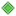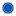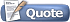View Single Post03-4-2019, 11:53 PM   #21
reuben_tate
Kawaii Desu Ne?Join Date: Dec 2007
Location: The Kawaiian Island~
Age: 28
Posts: 4,131Re: Petition to rename Chit Chat to Chitter Chatter

Quote:
 Originally Posted by floatiestringLet xn be the sum of all complex roots to the polynomial equation x^n = 1. Then, let a be the arithmetic mean of all xn for integer n. I give this petition a resounding +a.
When n = 0, we have infinitely many solutions to x^n = 1, so how you define x_0 in this case (if you do it as an infinite sum, in what order are the summands?)

Also, when you say "let a be the arithmetic mean of all x_n for integer n" it seems like you're taking the mean of an infinite set. How are you defining this exactly?
__________________